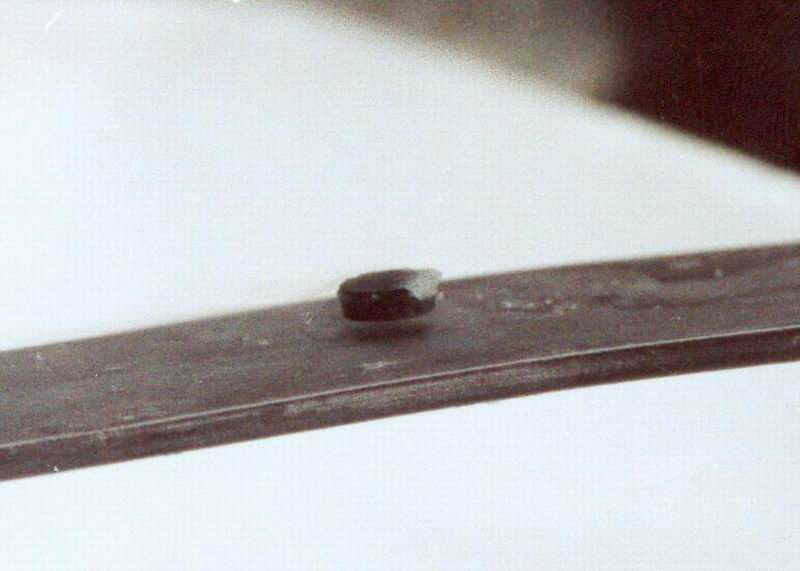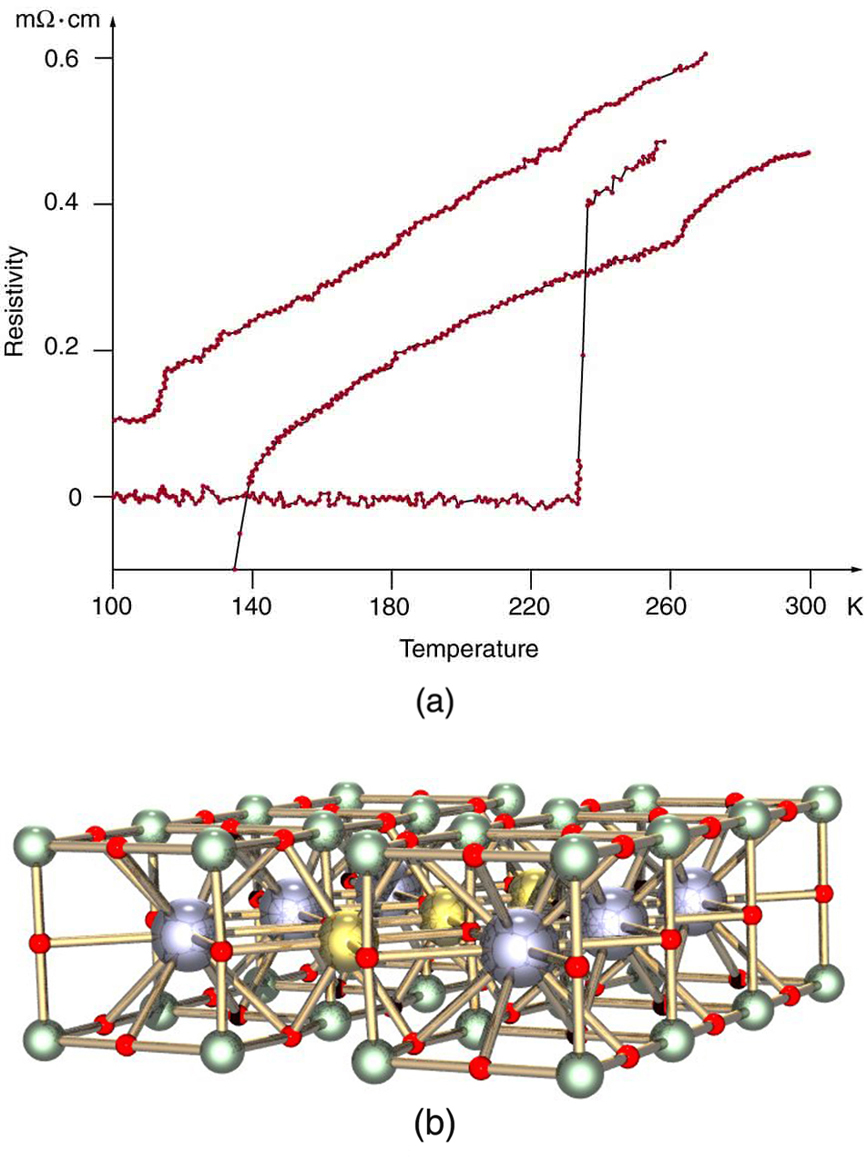# 34.6 High-temperature superconductors  (Page 2/4)

 Page 2 / 4One characteristic of a superconductor is that it excludes magnetic flux and, thus, repels other magnets. The small magnet levitated above a high-temperature superconductor, which is cooled by liquid nitrogen, gives evidence that the material is superconducting. When the material warms and becomes conducting, magnetic flux can penetrate it, and the magnet will rest upon it. (credit: Saperaud)

The search is on for even higher ${T}_{c}$ superconductors, many of complex and exotic copper oxide ceramics, sometimes including strontium, mercury, or yttrium as well as barium, calcium, and other elements. Room temperature (about 293 K) would be ideal, but any temperature close to room temperature is relatively cheap to produce and maintain. There are persistent reports of ${T}_{c}$ s over 200 K and some in the vicinity of 270 K. Unfortunately, these observations are not routinely reproducible, with samples losing their superconducting nature once heated and recooled (cycled) a few times (see [link] .) They are now called USOs or unidentified superconducting objects, out of frustration and the refusal of some samples to show high ${T}_{c}$ even though produced in the same manner as others. Reproducibility is crucial to discovery, and researchers are justifiably reluctant to claim the breakthrough they all seek. Time will tell whether USOs are real or an experimental quirk.

The theory of ordinary superconductors is difficult, involving quantum effects for widely separated electrons traveling through a material. Electrons couple in a manner that allows them to get through the material without losing energy to it, making it a superconductor. High- ${T}_{c}$ superconductors are more difficult to understand theoretically, but theorists seem to be closing in on a workable theory. The difficulty of understanding how electrons can sneak through materials without losing energy in collisions is even greater at higher temperatures, where vibrating atoms should get in the way. Discoverers of high ${T}_{c}$ may feel something analogous to what a politician once said upon an unexpected election victory—“I wonder what we did right?”(a) This graph, adapted from an article in Physics Today , shows the behavior of a single sample of a high-temperature superconductor in three different trials. In one case the sample exhibited a T c size 12{T rSub { size 8{c} } } {} of about 230 K, whereas in the others it did not become superconducting at all. The lack of reproducibility is typical of forefront experiments and prohibits definitive conclusions. (b) This colorful diagram shows the complex but systematic nature of the lattice structure of a high-temperature superconducting ceramic. (credit: en:Cadmium, Wikimedia Commons)

## Section summary

• High-temperature superconductors are materials that become superconducting at temperatures well above a few kelvin.
• The critical temperature ${T}_{c}$ is the temperature below which a material is superconducting.
• Some high-temperature superconductors have verified ${T}_{c}$ s above 125 K, and there are reports of ${T}_{c}$ s as high as 250 K.

## Conceptual questions

What is critical temperature ${T}_{c}$ ? Do all materials have a critical temperature? Explain why or why not.

Explain how good thermal contact with liquid nitrogen can keep objects at a temperature of 77 K (liquid nitrogen’s boiling point at atmospheric pressure).

Not only is liquid nitrogen a cheaper coolant than liquid helium, its boiling point is higher (77 K vs. 4.2 K). How does higher temperature help lower the cost of cooling a material? Explain in terms of the rate of heat transfer being related to the temperature difference between the sample and its surroundings.

## Problem exercises

A section of superconducting wire carries a current of 100 A and requires 1.00 L of liquid nitrogen per hour to keep it below its critical temperature. For it to be economically advantageous to use a superconducting wire, the cost of cooling the wire must be less than the cost of energy lost to heat in the wire. Assume that the cost of liquid nitrogen is $0.30 per liter, and that electric energy costs$0.10 per kW·h. What is the resistance of a normal wire that costs as much in wasted electric energy as the cost of liquid nitrogen for the superconductor?

$\text{0.30 Ω}$

#### Questions & Answers

What does mean ohms law imply
what is matter
Anything that occupies space
Kevin
Any thing that has weight and occupies space
Victoria
Anything which we can feel by any of our 5 sense organs
Suraj
Right
Roben
thanks
Suraj
what is a sulphate
Alo
Alo
the time rate of increase in velocity is called
acceleration
Emma
What is uniform velocity
Victoria
Greetings,users of that wonderful app.
how to solve pressure?
how do we calculate weight and eara eg an elefant that weight 2000kg has four fits or legs search of surface eara is 0.1m2(1metre square) incontact with the ground=10m2(g =10m2)
Cruz
P=F/A
Mira
can someone derive the formula a little bit deeper?
Bern
what is coplanar force?
what is accuracy and precision
How does a current follow?
follow?
akif
which one dc or ac current.
akif
how does a current following?
Vineeta
?
akif
AC current
Vineeta
AC current follows due to changing electric field and magnetic field.
akif
you guys are just saying follow is flow not follow please
Abubakar
ok bro thanks
akif
flows
Abubakar
but i wanted to understand him/her in his own language
akif
but I think the statement is written in English not any other language
Abubakar
my mean that in which form he/she written this,will understand better in this form, i write.
akif
ok
Abubakar
ok thanks bro. my mistake
Vineeta
u are welcome
Abubakar
what is a semiconductor
substances having lower forbidden gap between valence band and conduction band
akif
what is a conductor?
Vineeta
replace lower by higher only
akif
convert 56°c to kelvin
Abubakar
How does a current follow?
Vineeta
A semiconductor is any material whose conduction lies between that of a conductor and an insulator.
AKOWUAH
what is Atom? what is molecules? what is ions?
What is a molecule
Is a unit of a compound that has two or more atoms either of the same or different atoms
Justice
A molecule is the smallest indivisible unit of a compound, Just like the atom is the smallest indivisible unit of an element.
Rachel
what is a molecule?
Vineeta
what is a vector
A quantity that has both a magnitude AND a direction. E.g velocity, acceleration, force are all vector quantities. Hope this helps :)
deage
what is the difference between velocity and relative velocity?
Mackson
Velocity is the rate of change of displacement with time. Relative velocity on the other hand is the velocity observed by an observer with respect to a reference point.
Chuks
what do u understand by Ultraviolet catastrophe?
Rufai
A certain freely falling object, released from rest, requires 1.5seconds to travel the last 30metres before it hits the ground. (a) Find the velocity of the object when it is 30metres above the ground.
Mackson
A vector is a quantity that has both magnitude and direction
Rufus
the velocity Is 20m/s-2
Rufus
derivation of electric potential
V = Er = (kq/r^2)×r V = kq/r Where V: electric potential.
Chuks
what is the difference between simple motion and simple harmonic motion ?
syed
hi
Peace
hi
Rufus
hi
Chip
simple harmonic motion is a motion of tro and fro of simple pendulum and the likes while simple motion is a linear motion on a straight line.
Muinat
a body acceleration uniform from rest a 6m/s -2 for 8sec and decelerate uniformly to rest in the next 5sec,the magnitude of the deceleration is ?
The wording not very clear kindly
Moses
6
Leo
9.6m/s2
Jolly
the magnitude of deceleration =-9.8ms-2. first find the final velocity using the known acceleration and time. next use the calculated velocity to find the size of deceleration.
Mackson
wrong
Peace
-3.4m/s-2
Justice
Hi
Abj
Firstly, calculate final velocity of the body and then the deceleration. The final ans is,-9.6ms-2
Muinat
8x6= 48m/-2 use v=u + at 48÷5=9.6
Lawrence
can i define motion like this motion can be define as the continuous change of an object or position
Any object in motion will come to rest after a time duration. Different objects may cover equal distance in different time duration. Therefore, motion is defined as a change in position depending on time.
ChuksByByByByByBy